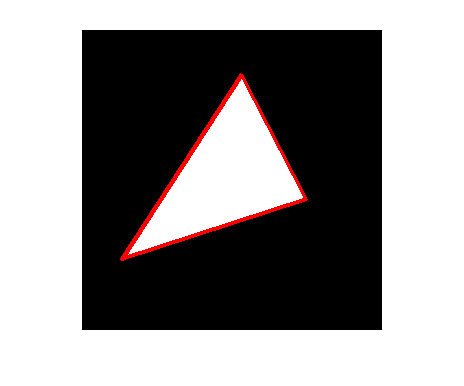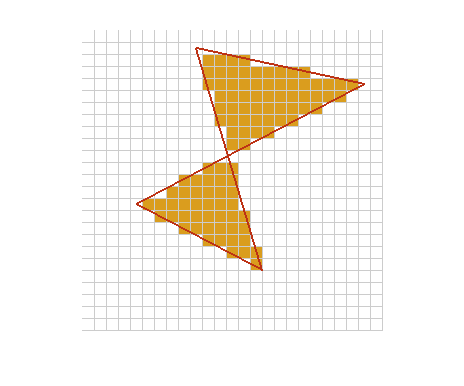# POLY2MASK and ROIPOLY – Part 119

Posted by Steve Eddins,

This is the first of several blogs about the algorithm behind poly2mask and roipoly. poly2mask takes a set of polygon vertices and produces a binary mask image. The binary mask has 1s for pixels inside the polygon and 0s for pixels outside.

Here's a quick peek at what it does.

x1 = [40 225 160 40];
y1 = [230 170 45 230];
hold on
plot(x1,y1,'LineWidth',3,'Color','r');
hold offEarlier versions of the Image Processing Toolbox just had roipoly. It contained both the core algorithm as well as a simple GUI for specifying the polygon vertices with mouse clicks. A few releases ago, we separated the core algorithm into its own function, poly2mask. Now roipoly handles image display and grabbing mouse clicks, and it calls poly2mask for the computation.

You can see that the computation might be nontrivial if you use a more complicated polygon, and if you zoom in a bit so you can see what's going on along the polygon edges. My second example computes a 25-by-25 mask, displays the mask using high magnification, and displays a gray grid showing the edges of the pixels.

x2 = [10 24 5 15.5 10];
y2 = [2 5 15 20.5 2];
M = 25;
N = 25;

map = [255 255 255; 218 157 30] / 255;

hold on
xx = 0.5:1:N+0.5;
yy = 0.5:1:M+0.5;
for k = 1:numel(xx)
plot([xx(k) xx(k)], [0.5 M+0.5], 'Color', [.8 .8 .8]);
end

for k = 1:numel(yy)
plot([0.5 N+0.5], [yy(k) yy(k)], 'Color', [.8 .8 .8]);
end

plot(x2,y2,'LineWidth',2,'Color',[189 50 21]/255);
hold offThe interesting pixels are those which are only partially inside the polygon. How do we decide the mask value for those pixels?

In the computer graphics space, I believe the most common term for this operation is polygon scan conversion. If you do a Web search on this term you'll find lots of links, many of which go to computer graphics course notes.

My own educational background is in electrical engineering, specializing in digital image and signal processing. When I left academia to become a software developer, implementing image processing algorithms, I found that many things I really needed to know were in the computer graphics literature, not in the image processing literature. (That's why the image processing team has Graphics Gems volumes 1-5 on our bookshelf.) That is most certainly the case for polygon scan conversion.

In my next blogs on this topic, I'll explain the desirable properties that a polygon scan conversion algorithm should have, the special cases that tend to trip up simple implementations, and the details of the Image Processing Toolbox implementation.

Get the MATLAB code

Published with MATLAB® 7.3

45 views (last 30 days)  | |